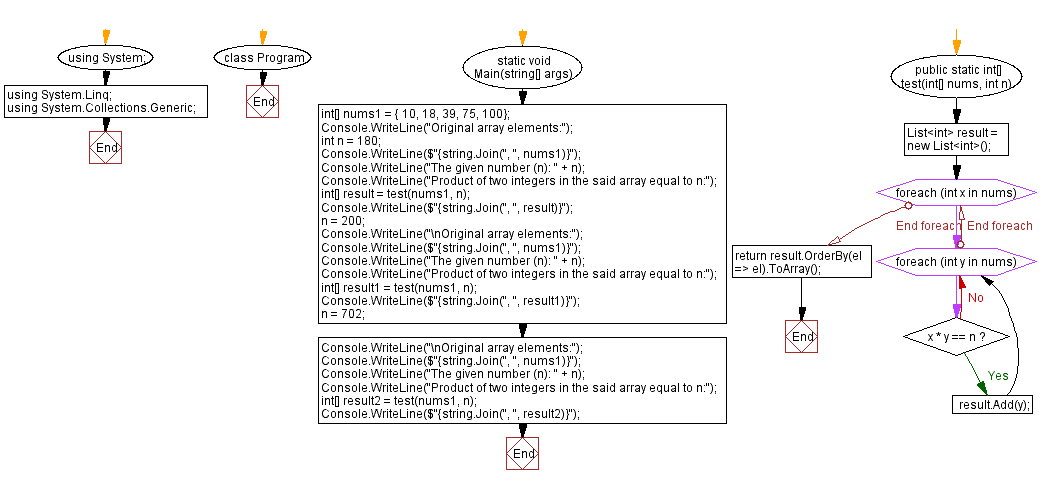﻿ C# - Find the product of two integers in an array# C# Sharp Exercises: Find the product of two integers in an array

## C# Sharp Array: Exercise-41 with Solution

Write a C# Sharp program to find two numbers in an array of integers whose product is equal to a given number.

Sample Data:
({10, 18, 39, 75, 100}, 180) -> {10, 18}
({10, 18, 39, 75, 100}, 200) -> {}
({10, 18, 39, 75, 100}, 702) -> {18, 39}

Sample Solution:

C# Sharp Code:

``````using System;
using System.Linq;
using System.Collections.Generic;namespace exercises
{
class Program
{
static void Main(string[] args)
{
int[] nums1 = { 10, 18, 39, 75, 100};
Console.WriteLine("Original array elements:");
int n = 180;
Console.WriteLine(\$"{string.Join(", ", nums1)}");
Console.WriteLine("The given number (n): " + n);
Console.WriteLine("Product of two integers in the said array equal to n:");
int[] result = test(nums1, n);
Console.WriteLine(\$"{string.Join(", ", result)}");
n = 200;
Console.WriteLine("\nOriginal array elements:");
Console.WriteLine(\$"{string.Join(", ", nums1)}");
Console.WriteLine("The given number (n): " + n);
Console.WriteLine("Product of two integers in the said array equal to n:");
int[] result1 = test(nums1, n);
Console.WriteLine(\$"{string.Join(", ", result1)}");
n = 702;
Console.WriteLine("\nOriginal array elements:");
Console.WriteLine(\$"{string.Join(", ", nums1)}");
Console.WriteLine("The given number (n): " + n);
Console.WriteLine("Product of two integers in the said array equal to n:");
int[] result2 = test(nums1, n);
Console.WriteLine(\$"{string.Join(", ", result2)}");       }
public static int[] test(int[] nums, int n)
{
List<int> result = new List<int>();
foreach (int x in nums)
{
foreach (int y in nums)
{
if (x * y == n)
{
}
}
}
return result.OrderBy(el => el).ToArray();
}
}
}
```
```

Sample Output:

```Original array elements:
10, 18, 39, 75, 100
The given number (n): 180
Product of two integers in the said array equal to n:
10, 18

Original array elements:
10, 18, 39, 75, 100
The given number (n): 200
Product of two integers in the said array equal to n:

Original array elements:
10, 18, 39, 75, 100
The given number (n): 702
Product of two integers in the said array equal to n:
18, 39
```

Flowchart:C# Sharp Code Editor:

Contribute your code and comments through Disqus.

Previous C# Sharp Exercise: Smallest positive which is not present in an array.
Next C# Sharp Exercise: C# Sharp Searching and Sorting Algorithm Exercises

What is the difficulty level of this exercise?

Test your Programming skills with w3resource's quiz.

﻿